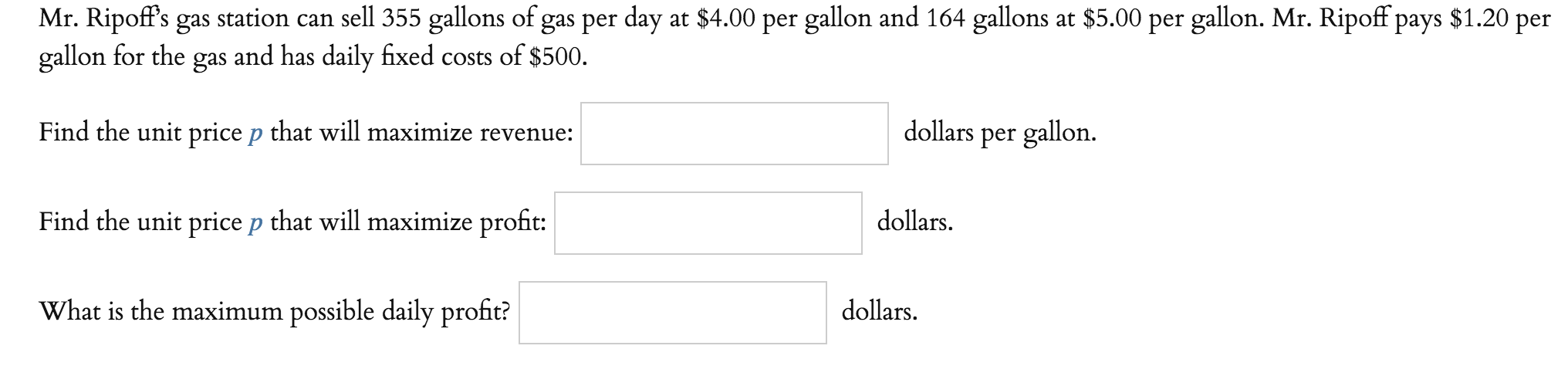# Mr. Ripoff's gas station can sell 355 gallons of gas per day at \$4.00 per gallon and 164 gallons at \$5.00 per gallon. Mr. Ripoff pays \$1.20 pergallon for the gas and has daily fixed costs of \$500.Find the unit price p that will maximize revenue:Find the unit price p that will maximize profit:What is the maximum possible daily profit?dollars per gallon.dollarsdollars.

Question
33 viewshelp_outlineImage TranscriptioncloseMr. Ripoff's gas station can sell 355 gallons of gas per day at \$4.00 per gallon and 164 gallons at \$5.00 per gallon. Mr. Ripoff pays \$1.20 per gallon for the gas and has daily fixed costs of \$500. Find the unit price p that will maximize revenue: Find the unit price p that will maximize profit: What is the maximum possible daily profit? dollars per gallon. dollars dollars. fullscreen
check_circle

star
star
star
star
star
1 Rating
Step 1

Let q be the gallons of gas sold per day.

Daily cost incurred C(q)=1.20q + 500.

Next we find the relationship between price at which gas is sold(p) and the quantity sold daily(q).

The relationship is linear and we are provided two points on the graph , namely, (355,4) and (164,5).

From this , the relation is determined as 191p+q=1119.

=>q=1119-191p

Revenue(R)=q*p=1119p-191p^2

Revenue will be maximun when dR/dp=0

Step 2

Calculating dR/dp:

Step 3

i) For maximum Revenue, pric...

### Want to see the full answer?

See Solution

#### Want to see this answer and more?

Solutions are written by subject experts who are available 24/7. Questions are typically answered within 1 hour.*

See Solution
*Response times may vary by subject and question.
Tagged in

### Calculus